## PRACTICE PROBLEMS BASED ON TOKEN RING AND TOKEN PASSING-

Before you go through this article, make sure that you have gone through the previous article on Token Passing.

## Problem-01:

Token ring station operates in which of the following modes?

1. Transit mode
2. Listen mode
3. Bypass mode and Receive mode
4. All of the above

## Solution-

• In transmit mode, a station transmits the data.
• In listen mode, a station listens from other station(s).
• In bypass mode, a station simply bypasses the data packet if it is not meant for it.
• In receive mode, a station receives the data packet if it is destined to it.

Therefore, a token ring station operates in all these modes.

Thus, Option (D) is correct.

## Problem-02:

Efficiency of the token ring is high if-

1. Reinsert the token after receiving the last bit of the frame
2. Reinsert the token after receiving the last bit of the header
3. Reinsert the token after last bit of the data packet is transferred
4. Reinsert the token after last bit of the header is transferred

## Solution-

• There are two strategies used in token ring- Early Token Reinsertion (ETR) and Delayed Token Reinsertion (DTR).
• Efficiency of token ring is high in Early Token Reinsertion (ETR).

Thus, Option (C) is correct.

## Problem-03:

The sending station in IEEE 802.5 sets the address recognized (A) bit and frame copied (C) bit in MAC header as

1. 1,0
2. 0,0
3. 0,1
4. 1,1

## Solution-

• IEEE 802.5 is token ring.
• Sending station sets both the available bit and copied bit as 0.
• These bits are modified by the receiving station.
• If the receiving station is available, it sets the Available bit to 1.
• If the receiving station successfully copies the data, it sets the Copied bit to 1.

Thus, Option (B) is correct.

## Problem-04:

Which of the following fields in 802.5 MAC header is not included in CRC or FCS?

1. FC
2. Data field
3. FS
4. SA

## Solution-

• IEEE 802.5 is token ring.
• Frame Status (FS) field consists of the Available bit and Copied bit.
• These two bits are modified by the receiving station.
• So, CRC is not computed on Frame Status field otherwise receiving station will have to bear the overhead of recomputing the CRC.

Thus, Option (C) is correct.

## Problem-05:

What type of acknowledgement system is used in 802.5?

1. Cumulative ACK
2. Independent ACK
3. Piggybacking ACK
4. None

## Solution-

• IEEE 802.5 is token ring.
• The two bits- Available bit and Copied bit acts as the acknowledgement for the sending station.
• The value of these bits suggests to the sending station that whether the receiving station has successfully copied the data or not.
• Because the two bits are contained in the data frame, so we can say that piggybacked acknowledgements are used in token ring.

Thus, Option (C) is correct.

## Problem-06:

In token ring, ______ field is present only in the data / command frame but not in the token frame.

1. SD
2. AC
3. ED
4. FS

## Solution-

• Frame status (FS) field is present only in the data / command frame.
• A token frame consists of only 3 fields- SD, AC and ED.

Thus, Option (D) is correct.

## Problem-07:

Consider a token ring with latency 500 μsec and packet size of 1500 bytes. What is the effective throughput rate for both single active host and for many active hosts that can be achieved if the ring has 3 Mbps bandwidth? Assume the strategy used is delayed token reinsertion.

1. 2.4 Mbps and 3 Mbps
2. 2.4 Mbps and 2 Mbps
3. 2 Mbps and 3 Mbps
4. 2.4 Mbps and 2.67 Mbps

## Solution-

Given-

• Ring latency = 500 μsec
• Packet Size = 1500 bytes
• Bandwidth = 3 Mbps
• Strategy used is Delayed Token Reinsertion (DTR)

Efficiency of Delayed Token Reinsertion (DTR) strategy is-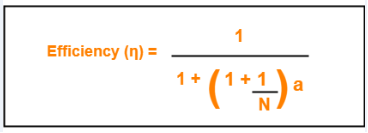## Calculating Transmission delay-

We know,

Transmission delay (Tt)

= Packet size / Bandwidth

= 1500 bytes / 3 Mbps

= (1500 x 8 bits) / (3 x 106 bits per sec)

= 4000 μsec

## Calculating value of ‘a’-

We know,

a = Tp / Tt

a = Latency / Tt

a = 500 μsec / 4000 μsec

a = 0.125

## Calculating Throughput for single active host-

For single active host, N = 1.

Substituting N = 1 in efficiency formula, we get-

Efficiency (η)

= 1 / (1 + 2a)

= 1 / (1 + 2 x 0.125)

= 1 / 1.25

= 0.8

Now,

Throughput

= Efficiency (η) x Bandwidth

= 0.8 x 3 Mbps

= 2.4 Mbps

## Calculating Throughput for many active host-

For many active host, N = ∞.

Substituting N = ∞ in efficiency formula, we get-

Efficiency (η)

= 1 / (1 + a)

= 1 / (1 + 0.125)

= 1 / 1.125

= 0.89

Now,

Throughput

= Efficiency (η) x Bandwidth

= 0.89 x 3 Mbps

= 2.67 Mbps

Thus, Option (D) is correct.

## Problem-08:

In 802.5, the condition to find out the minimum size of the ring is-

1. Latency of the ring = Transmission delay of the data frame
2. Latency of the ring = Transmission delay of the token frame
3. Latency of the ring = RTT
4. Latency > RTT

## Solution-

• IEEE 802.5 is token ring.
• The condition to find out the minimum size of the ring is-

Latency of the ring >= Transmission delay of the token frame

• In worst case, all the stations goes down and only the monitor station is alive.
• Monitor station sends the token and the token comes back to it.
• To avoid the collision between the first and the last bit of the token, propagation delay of the token must be at least equal to its transmission delay.

Thus, Option (B) is correct.

## Problem-09:

The stacking station is a station in 802.5 and it can be described as-

1. when it drains the frame and creates a token, it then stores both the old and new priority of the token
2. A station which stack the token till it gets the last bit of the token
3. It is nothing but monitor station
4. None of the above

## Solution-

• IEEE 802.5 is token ring.
• A station which increases the priority of the token should next decrease the priority of the token.
• Otherwise once the priority of the token reaches the highest value, it would ever remain there and thus only the station with the highest packet would drain (take) the token and use it.
• Thus, a stacking station must remember both the old and new priorities so that when it later receives the token with new priority, it changes it to old priority.
• This is implemented by using 2 stacks. Each station maintains 2 stacks where one station keeps track of the old priority and other stack keeps track of the new priority.

Thus, Option (A) is correct.

## Problem-10:

In early token release, the station releases a token as soon as it completes the frame transmission whether or not the frame header has returned to the station, then what will be the priority of the token released?

1. The priority in the frame that completes frame transmission
2. Without changing the priority, token will be released
3. Default priority is used
4. The priority in the reservation field of the most recently received frame

## Solution-

Option (D) is correct.

## Problem-11:

Find the efficiency of the ring where data rate of the link is 4 Mbps, number of stations are 20, separated by 100 meters and bit delay in each station is 2.5 bits. Assume early token reinsertion with packet size of 1000 bits and transmission speed is 2 x 108 m/sec.

## Solution-

Given-

• Data rate = Bandwidth = 4 Mbps
• Number of stations = 20
• Distance between two stations = 100 meters
• Bit delay = 25 bits
• Packet size = 1000 bits
• Strategy used is Early Token Reinsertion (ETR)

### Calculating length of ring wire-

Total length of ring wire

= Number of stations x Distance between 2 stations

= 20 x 100 meters

= 2000 meters

= 2 km

### Calculating Transmission delay-

Transmission delay (Tt)

= Packet size / Bandwidth

= 1000 bits / 4 Mbps

= 1000 bits / (4 x 106 bits per sec)

= 250 μsec

### Calculating Propagation delay-

Propagation delay (Tt)

= Distance / Speed

= 2 km / (2 x 108 m/sec)

= (2 x 103 m) / (2 x 108 m/sec)

= 10-5 sec

= 10 μsec

### Calculating Bit delay in seconds-

Bit delay

= 25 bits

= 2.5 bits / 4 Mbps

= 25 bits / (4 x 106 bits per sec)

= 0.625 μsec

### Calculating Ring latency-

Ring latency

= Propagation delay + N x Bit delay

= 10 μsec + 20 x 0.625 μsec

= 10 μsec + 12.5 μsec

= 22.5 μsec

### Calculating value of ‘a’-

a

= Ring latency / Tt

= 22.5 μsec / 250 μsec

= 0.09

### Calculating Efficiency-

Efficiency(η)

= 1 / (1 + a/N)

= 1 / (1 + 0.09 / 20)

= 1 / 1.0045

= 0.9955

= 99.55%

## Problem-12:

A token ring LAN network interconnects M stations using Star Topology in the following way. All the input and output lines of the token ring station interface are connected to a cabinet where the actual ring is placed. Suppose that distance from each station to a cabinet is 100 m and ring latency per station is 8 bits, packets are 1250 B and bandwidth is 25 Mbps.

1. Find the ring latency normalized to packet transmission time.
2. Find the minimum number of packets transmitted by stations, if stations are allowed to transmit an unlimited number of packet / token. (v = 2 x 108 m/sec)

## Solution-

Based on the given information, the token ring LAN network looks like-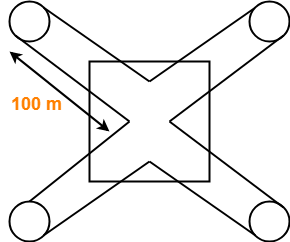(The sketch is for 4 stations)

### Calculating Transmission delay-

Transmission delay

= Packet size / Bandwidth

= 1250 B / 25 Mbps

= (1250 x 8 bits) / (25 x 106 bits per sec)

= 400 μsec

### Calculating Propagation delay-

Propagation delay

= Distance / Speed

= (200 x M meters) / (2 x 108 m/sec)

= (200 x M) / (2 x 108) sec

= 100 x M x 10-8 sec

= M μsec

### Calculating Bit delay in seconds-

Bit delay

= Ring latency per station

= 8 bits

= 8 bits / 25 Mbps

= 0.32 μsec

### Calculating Ring latency-

Ring latency

= Propagation delay + N x Bit delay

= M μsec + M x 0.32 μsec

= 1.32 x M μsec

### Calculating Ring latency normalized to packet transmission delay-

Ring latency normalized to packet transmission time

= Ring latency / Packet transmission time

= 1.32 x M μsec / 400 μsec

= 0.0033 x M

### Part-02:

• The number of packets a station can transmit after holding a token depends on Token Holding Time and the strategy used.
• Since no information is given in the question about the Time Holding Time, so we assume that there is no restriction on holding the token.
• Thus, a station can send infinite number of packets after getting a token.

## Problem-13:

A very heavily loaded 1 km long, 10 Mbps token ring has a propagation speed of 200 m/μsec. 50 stations are uniformly spaced around the ring. Data frames are 256 bits including 32 bits of overhead. Acknowledgements are piggybacked onto the data frames and are thus included as spare bits within the data frames and are effectively free. The token is 8 bits long. Is the effective data rate of this ring higher or lower than the effective data rate of a 10 Mbps CSMA / CD network? Assume ‘Early Token Release’ policy.

## Solution-

### Remember

Token Ring always beats the Ethernet in terms of effective bandwidth.

### Efficiency of CSMA / CD-

Efficiency of token ring in early token retransmission is given by-

Efficiency(η) = 1 / (1 + 6.44 x a)

This expression is valid when number of stations are very large i.e. N → ∞

### Case-01:

Efficiency of token ring in early token retransmission is given by-

Efficiency(η) = 1 / (1 + a/N)

When N → ∞, Efficiency(η) = 100%

### Case-02:

Efficiency of token ring in delayed token retransmission is given by-

Efficiency(η) = 1 / [1 + a(1 + 1/N)]

When N → ∞, Efficiency(η) = 1 / (1+a)

The above analysis clearly shows-

Efficiency of Token Ring in ETR > Efficiency of Token Ring in DTR > Efficiency of Token Ring in CSMA / CD.

## Problem-14:

A fibre optic token ring used as a MAN is 200 km long and runs at 100 Mbps. After sending a frame, a station drains the frame from the ring before regenerating the token. The signal propagation speed in the fibre is 200,000 km/sec and maximum frame size is 1 KB. What is the maximum efficiency at N=1?

## Solution-

Given-

• Distance = 200 km
• Bandwidth = 100 Mbps
• Propagation speed = 200,000 km/sec = 2 x 108 m/sec
• Frame size = 1 KB
• Number of stations = 1
• Strategy used is Delayed Token Reinsertion

### Calculating Transmission delay-

Transmission delay

= Frame size / Bandwidth

= 1 KB / 100 Mbps

= (1 x 210 x 8 bits) / (100 x 106 bits per sec)

= 81.92 μsec

### Calculating Propagation delay-

Propagation delay

= Distance / Speed

= 200 km / (2 x 108 m/sec)

= (200 x 103 m) / (2 x 108 m/sec)

= 10-3 sec

= 1 msec

### Calculating value of ‘a’-

a

= Tp / Tt

= 1 msec / 81.92 μsec

= 0.0122 x 103

= 12.2

### Calculating Efficiency-

Efficiency(η)

= 1 / [1 + a x (1+ 1/N)]

= 1 / [1 + 12.2 x (1+1)]

= 1 / 25.4

= 0.0394

= 3.94%

• The reason behind this much less efficiency is that the distance is too large here.
• Ethernet and Token Ring are meant for LANs.
• If used for MANs or WANs, the efficiency will fall drastically.

## Problem-15:

At a propagation speed of 200 m/μsec, what is the effective length added to a ring by a bit delay at each repeater or station for-

1. 1 Mbps line
2. 40 Mbps line

## Solution-

### Part-01:

Effective length added to a ring by a bit delay

= 1 bit / 1 Mbps

= 1 μsec

= 1 μsec x 200 m/μsec

= 200 m

### Part-02:

Effective length added to a ring by a bit delay

= 1 bit / 40 Mbps

= 0.025 μsec

= 0.025 μsec x 200 m/μsec

= 5 m

## Problem-16:

Consider a 10 Mbps token ring LAN with a ring latency of 400 μs. A host that needs to transmit seizes the toke. Then it sends a frame of 1000 bytes, removes the frame after it has circulated all around the ring and finally releases the token. This process is repeated for every frame. Assuming that only a single host wishes to transmit, the effective data rate is _____ .

1. 1 Mbps
2. 2 Mbps
3. 5 Mbps
4. 6 Mbps

## Solution-

Given-

• Bandwidth = 10 Mbps
• Ring latency = 400 μsec
• Frame size = 1000 bytes
• Number of stations = 1
• Strategy used is Delayed Toke Reinsertion

### Calculating Transmission delay-

Transmission delay

= Frame size / Bandwidth

= 1000 bytes / 10 Mbps

= (1000 x 8 bits) / (10 x 106 bits per sec)

= 800 μsec

### Calculating value of ‘a’-

a

= Ring latency / Tt

= 400 μsec / 800 μsec

= 0.5

### Calculating Efficiency-

Efficiency(η)

= 1 / [1 + a x (1+ 1/N)]

= 1 / [1 + 0.5 x (1+1)]

= 1 / 2

= 0.50

= 50%

### Calculating Effective data rate-

Effective data rate

= Throughput

= Efficiency(η) x Bandwidth

= 0.5 x 10 Mbps

= 5 Mbps

Thus, Option (C) is correct.

Next Article-Aloha | Pure Aloha | Slotted Aloha

Get more notes and other study material of Computer Networks.

Watch video lectures by visiting our YouTube channel LearnVidFun.

## Access Control in Networking-

Before you go through this article, make sure that you have gone through the previous article on Access Control.

We have discussed-

• Access Control is a mechanism that controls the access of stations to the transmission link.
• There are various access control methods-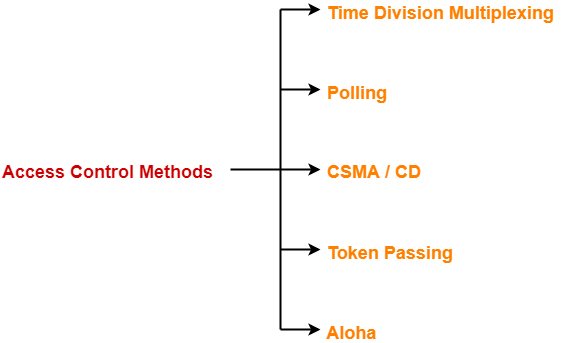Before discussing Token Passing, let us discuss few important concepts required for the discussion.

## Time Conversions-

In token passing,

• Time may be expressed in seconds, bits or meters.
• To convert the time from one unit to another, we use the following conversion chart-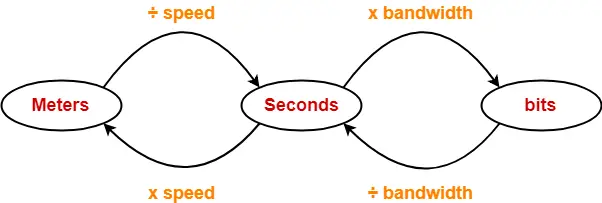## Token Passing Terminology-

The following terms are frequently used-

1. Token
2. Ring Latency
3. Cycle Time

## 1. Token-

• A token is a small message composed of a special bit pattern.
• It represents the permission to send the data packet.
• A station is allowed to transmit a data packet if and only if it possess the token otherwise not.

## 2. Ring Latency-

 Time taken by a bit to complete one revolution of the ring is called as ring latency.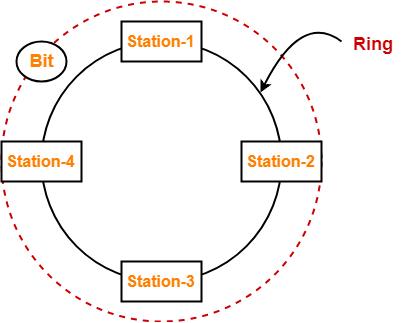Let us derive the expression for ring latency.

If-

• Length of the ring = d
• Speed of the bit = v
• Number of stations = N
• Bit delay at each station = b

(Bit delay is the time for which a station holds the bit before transmitting to the other side)

Then-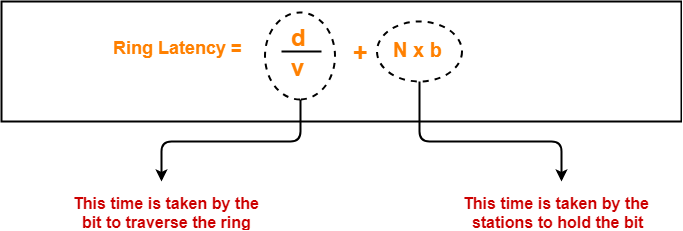## Notes-

• d / v is the propagation delay (Tp) expressed in seconds.
• Generally, bit delay is expressed in bits.
• So, both the terms (d / v and N x b) have different units.
• While calculating the ring latency, both the terms are brought into the same unit.
• The above conversion chart is used for conversion.

After conversion, we have-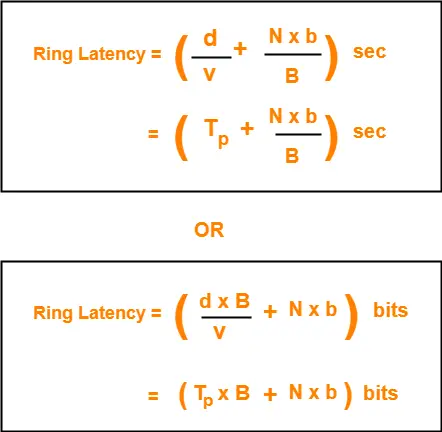## 3. Cycle Time-

 Time taken by the token to complete one revolution of the ring is called as cycle time.

If-

• Length of the ring = d
• Speed of the bit = v
• Number of stations = N
• Token Holding Time = THT

(Token Holding Time is the time for which a station holds the token before transmitting to the other side)

Then-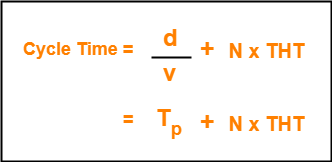Now, we start discussing about Token Passing Access Control Method.

## Token Passing-

In this access control method,

• All the stations are logically connected to each other in the form of a ring.
• The access of stations to the transmission link is governed by a token.
• A station is allowed to transmit a data packet if and only if it possess the token otherwise not.
• Each station passes the token to its neighboring station either clockwise or anti-clockwise.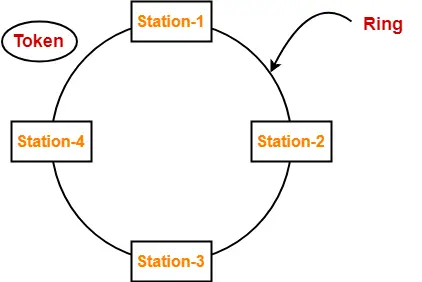## Assumptions-

Token passing method assumes-

• Each station in the ring has the data to send.
• Each station sends exactly one data packet after acquiring the token.

## Efficiency-

 Efficiency (η) = Useful Time / Total Time

In one cycle,

• Useful time = Sum of transmission delay of N stations since each station sends 1 data packet = N x Tt
• Total Time = Cycle time = Tp + N x THT

Thus,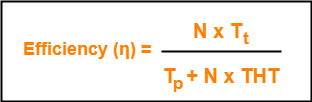Token Holding Time depends on the strategy implemented.

## Token Passing Strategies-

The following 2 strategies are used in token passing-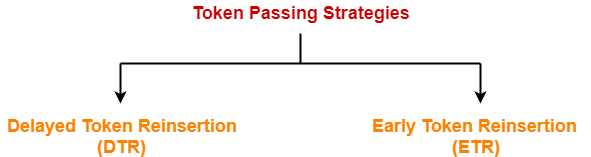1. Delayed Token Reinsertion (DTR)
2. Early Token Reinsertion (ETR)

## 1. Delayed Token Reinsertion-

In this strategy,

• Station keeps holding the token until the last bit of the data packet transmitted by it takes the complete revolution of the ring and comes back to it.

## Working-

After a station acquires the token,

• It transmits its data packet.
• It holds the token until the data packet reaches back to it.
• After data packet reaches to it, it discards its data packet as its journey is completed.
• It releases the token.

The following diagram illustrates these steps for station-1. Same procedure is repeated at every station.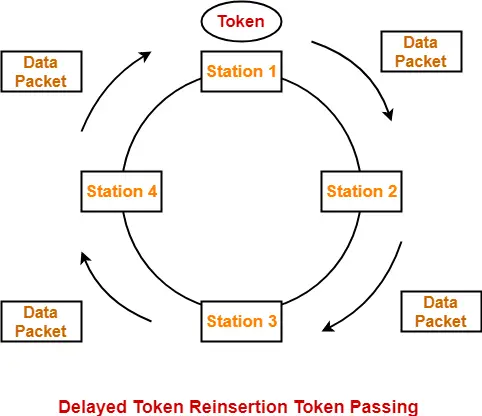## Token Holding Time-

 Token Holding Time (THT) = Transmission delay + Ring Latency

We know,

• Ring Latency = Tp + N x bit delay
• Assuming bit delay = 0 (in most cases), we get-

 Token Holding Time = Tt + Tp

## Efficiency-

Substituting THT = Tt + Tp in the efficiency expression, we get-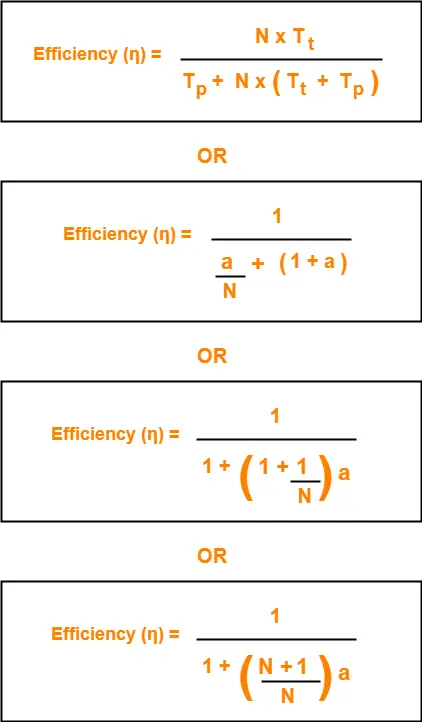## 2. Early Token Reinsertion-

In this strategy,

• Station releases the token immediately after putting its data packet to be transmitted on the ring.

## Working-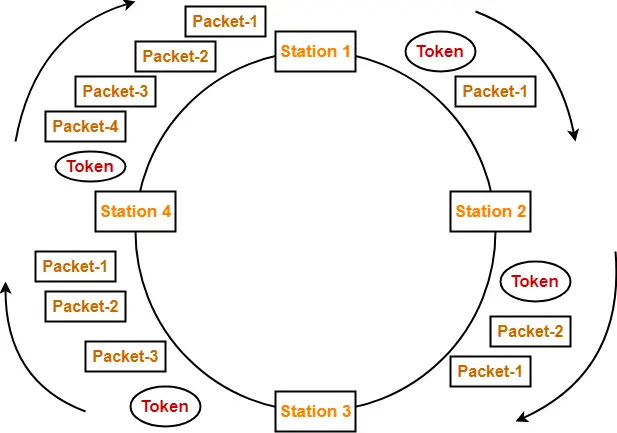### Step-01: At Station-1:

Station-1

• Acquires the token
• Transmits packet-1
• Releases the token

### Step-02: At Station-2:

Station-2

• Transmits packet-1
• Acquires the token
• Transmits packet-2
• Releases the token

### Step-03: At Station-3:

Station-3

• Transmits packet-1
• Transmits packet-2
• Acquires the token
• Transmits packet-3
• Releases the token

### Step-04: At Station-4:

Station-4

• Transmits packet-1
• Transmits packet-2
• Transmits packet-3
• Acquires the token
• Transmits packet-4
• Releases the token

### Step-05: At Station-1:

• Discards packet-1 (as its journey is completed)
• Transmits packet-2
• Transmits packet-3
• Transmits packet-4
• Acquires the token
• Transmits packet-1 (new)
• Releases the token

In this manner, the cycle continues.

## Token Holding Time-

 Token Holding Time (THT) = Transmission delay of data packet = Tt

## Efficiency-

Substituting THT = Tt in the efficiency expression, we get-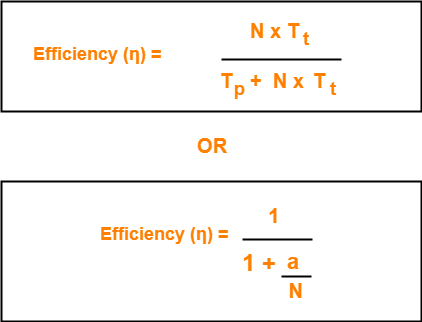## Differences between DTR and ETR-

 Delay Token Retransmission (DTR) Early Token Retransmission (ETR) Each station holds the token until its data packet reaches back to it. Each station releases the token immediately after putting its data packet on the ring. There exists only one data packet on the ring at any given instance. There exists more than one data packet on the ring at any given instance. It is more reliable than ETR. It is less reliable than DTR. It has low efficiency as compared to ETR. It has high efficiency as compared to ETR.

## Note-01:

In token passing,

• It is the responsibility of each transmitting station to remove its own data packet from the ring.

## Note-02:

While solving questions,

• If the strategy used is not mentioned, then consider Early Token Retransmission strategy.

To gain better understanding about Token Passing Method,

Watch this Video Lecture

Next Article-Practice Problems On Token Passing

Get more notes and other study material of Computer Networks.

Watch video lectures by visiting our YouTube channel LearnVidFun.